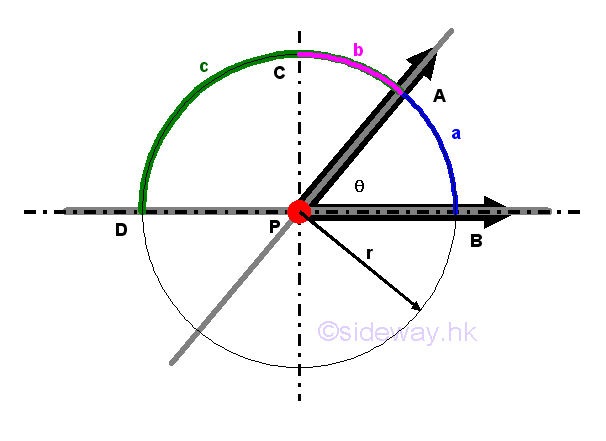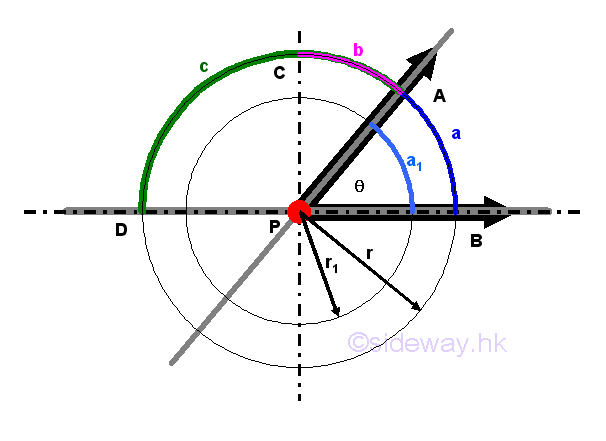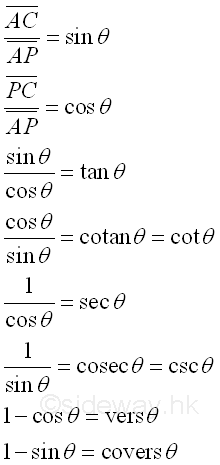output.to from Sideway
Draft for Information Only

# Content

`  Trigonometry   Angle   Functions of an Angle   Relations of Functions of an Angle`

## Trigonometry

Trigonometry is originally the study of the correlation between the sides and angles of a triangle. Trigonometry is also called plane trigonometry because the triangle is described on a plane. When the triangle is described on a spherical surface, it is called spherical trigonometry.

### Angle

An angle is defined as the inclination of one straight line to another straight line about a point in geometry but a more boarder meaning is used in trigonometry where angle is the revolution of a ray refered to a fixed ray with a common vertex and the angular motion can be either counter clockwise or clockwise. Angle measurement in trigonometry is therefore can be greater than π or 2π in general.Let θ be the angle of APB in radian and a be the subtending arc by angle θ at radius r. Let π be the straight angle. Then angle (π/2-θ) is called the complement of θ and angle (π-θ) is called the supplement of θ. Similarly arc b is called the complement of a and arc c is called the supplement of a.From geometry, the ratio of the circumference of any circle over its radius is equal to a constant quantity 2π. Since angle at C is proportional to the subtending arc. Therefore an angle can be expressed in term of the arc subtended by proportional,  rθ/2πr=θ/2π. When the arc length is equal to the radius, by proportional, r/2πr=1/2π, the quantity from radius is called  one radian. A right angle is equal to π/2 and a straight angle is equal to π.

Another way to measure an angle is by dividing the circumference of a complete circle into 360 equal portions. The angle subtended by one portion of the divided arc is equal to one degree. Each degree can be divided into 60 equal parts call minutes. Each minute can further be divided into 60 equal parts call seconds. A right angle is therefore equal to 90 degrees and a straight angle is equal to 180 degrees. The division of circumference of a circle into 360 degrees is called the sexagesimal divison which is difference from those division by dividing a circle into 100 degress called centesimal.

Since both radian and degree are dervied from the circumference of a circle, both measurements can be converted to each other through a simple relationship, i.e. degree/360=radian/2π.

The division of the circumference of a circle into 360 degrees with 1 degree equals to 60 minutes and 1 minute equals to 60 seconds is called sexagesimal division. And there is also an impopular centesimal division, for which the circumference of a circle into 100 degrees with 1 degree equals to 100 minutes and 1 minute equals to 100 seconds

### Functions of an AngleLet P be the centre of a circle of any radius, PB be a horizontal line and θ be the angle subtended by an arc BA with sides PB and PA. A line AC is drawed perpendicular to PB. The ratio of AC over PA is called the sine of the angle θ and the ratio of PC over PA is called the cosine of the angle θ. The ratio of sine of the angle θ to the cosine of the angle θ is called the tangent of angle θ, while the inverse of the ratio is called the cotangent. The ratio of unity to the cosine of angle θ is called the secant of angle θ, and the ratio of unity to the sine of angle θ is called cosecant of angle θ. The difference between unity and the cosine of angle θ is called the versed-sine of angle θ, the difference between unity and the sine of angle θ is called the coversed-sine of angle θ. Imply functions of angle are### Relations of Functions of an Angle

From the right triangle ACP, by Pythagorean theorem

©sideway

ID: 130700004 Last Updated: 7/2/2013 Revision: 0 Ref:References

1. B. Joseph, 1978, University Mathematics: A Textbook for Students of Science &amp; Engineering
2. Ayres, F. JR, Moyer, R.E., 1999, Schaum's Outlines: Trigonometry
3. Hopkings, W., 1833, Elements of TrigonometryHome 5

Business

Management

HBR 3

Information

Recreation

Culture

Chinese 1097

English 337

Computer

Hardware 153

Software

Application 204

Latex 34

Manim 171

Numeric 19

Programming

Web 285

Unicode 504

HTML 65

CSS 59

ASP.NET 194

OS 412

Python 56

Knowledge

Mathematics

Algebra 30

Trigonometry 18

Geometry 21

Calculus 67

Engineering

Mechanical

Rigid Bodies

Statics 92

Dynamics 37

Control

Natural Sciences

Electric 27

Copyright © 2000-2021 Sideway . All rights reserved Disclaimers last modified on 06 September 2019